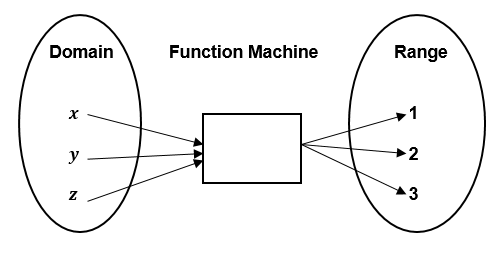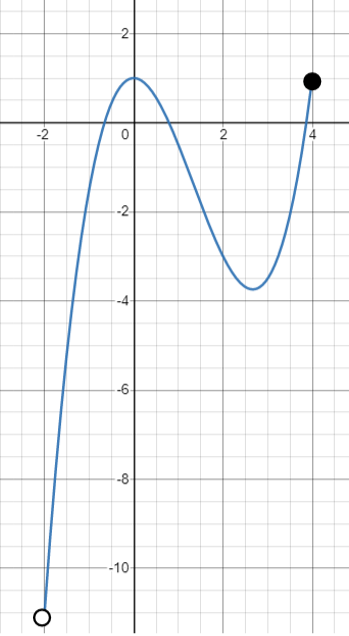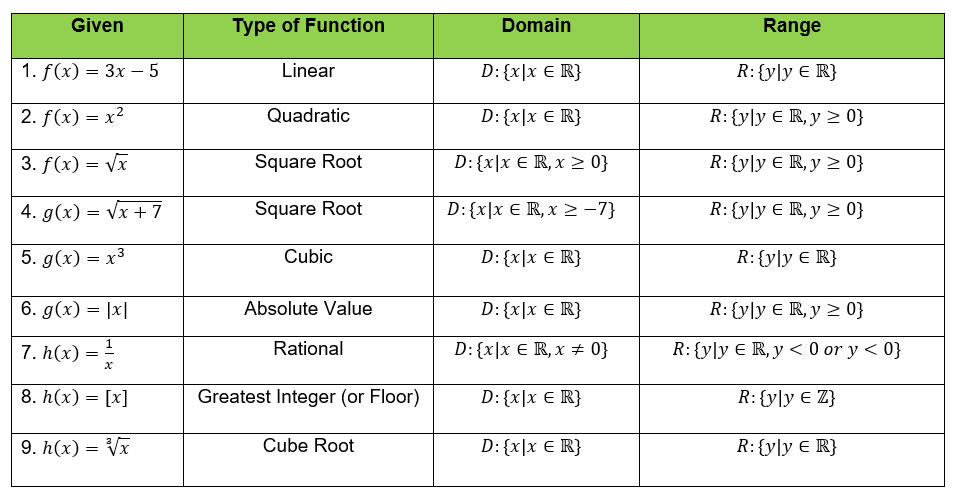407 493 6601

# 8th Grade - Domain and Range

## Introduction

• The domain of a relation is the set of first elements, while the range is the set of second coordinates.
• The domain is the complete set of all possible values of the independent variable $x$. It is the set of all inputs for a function or relation.
• The range is the complete set of all possible values of the dependent variable $y$. It is the set of all outputs for a function or relation.
• The range of a function is also called the image of the function.
• Since domain and range are sets, we can express them using the listing method, set-builder notation, or verbal form.
• For instance, the domain of a  set of all real numbers except $-5$ is written as .
• Other ways of writing the domain and range are inequalities and interval notation.
• The diagram below shows how a function relates two sets, the domain and range.## Finding the Domain and Range of a Relation

Ways to Write the Domain and Range

• Listing Method. In the relation example , we can write the domain and range using the listing method. The input values are the first coordinates in the ordered pairs. The domain is . The output values are the second coordinates in the ordered pairs. The range is .
• Verbal Form. In the example of the relation , we may write the domain and range in different ways. In verbal form, the domain is the set of all natural numbers. The range is the set of all positive perfect cube numbers.
• Set Builder Notation. This method of writing the set of elements has specific conditions that satisfy the relation or function. It uses the form  which is verbally expressed as a "set of all $x$ such that the condition for $x$ is true." For instance, we have .
• Interval Notation. It is a manner of writing sets that include all real numbers between a lower value that may or may not be included and an upper that may or may not be included in the set. A square bracket means a close interval, whereas a parenthesis means an open interval. For example, we have shown the set of all real numbers between 9 and 15 inclusive. In the case of , this implies all real numbers below $-3$ and above $2$.
• Inequalities. It is the method of writing sets that contain all real numbers between a minimum value and a maximum value. For instance, we have $-4. Other cases of inequalities are  and $1\le x\le 7$.

Finding the Domain and Range using graphs of functions and relations

• The domain of a graph consists of all input values, which are shown on the x-axis.
• The range of a graph consists of all output values, which are shown on the y-axis. Consider the minimum and maximum values of the function in the turning points of the graph.

Example: What is the domain of the function represented by its graph?Domain: $\left\{x|x\in \mathrm{ℝ},-2

Range: $\left\{y|y\in \mathrm{ℝ},-11

## Domain and Range of Some Functions

• Here are some basic or common functions with their respective domain and range.## Cheat Sheet

• The set of all real numbers is written in the following notations: or $\left\{x|x\in \mathrm{ℝ}\right\}$.
• The set of all integers is written as $\mathrm{ℤ}$ or $\left\{x|x\in \mathrm{ℤ}\right\}$.
• The set of all natural numbers (counting numbers) is written as $\mathrm{ℕ}$ or $\left\{x|x\in \mathrm{ℕ}\right\}$.
• When a relation or function is expressed in the table of values, mapping diagram, or set of ordered pairs, the domain is the set of all first elements (input values), and the range is the set of all second elements (output values).
• If a function is polynomial, the domain is the set of all real numbers. The range is also the set of all real numbers. Exclude all restrictions in the defining rule if there are any.
• In the case of $y=\sqrt{x-2}$, the domain is the set of all real numbers where $x-2\ge 0$.
• In the example of $y=-\left|x-1\right|$, the range is the set of all real numbers where $y\le 0$.
• In the case of $y=\frac{2}{x}$, the domain is the set of all real numbers where the denominator must not be equal to $0.$
• Aside from the domain and range, the concept of co-domain is also considered in higher mathematics disciplines.
• Co-Domain is the set from which the second elements are taken. In other words, it is where the range elements are obtained.

## Blunder Areas

• In writing the domain and range, be mindful of the restrictions in the graph, if there are any.
• An open circle in the graph of a relation or function indicates that the value is excluded in the domain or range.
• A closed circle in the graph of a relation or function indicates that the value is included in the domain or range.